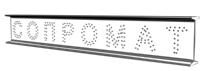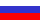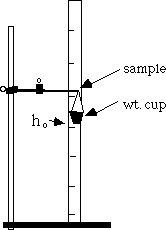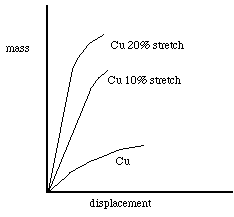# Strength of materials onlineBeam calculation (analysis) for strength online - curves Mx, Qy, Mtor, N, finding the maximal bending moment Mx, maximal moving force Qy. Statically indeterminable beams can be calculated too!!. All is easy! For Free!

FEA online. Truss, frame, beam analysis (calculation) for strength online - reactions, curves M, Q, M, N.

## Tensile Strength Test

Stretching Wires

Tensile Strength Test for Various Metals

Objective:

The objective of this experiment is to demonstrate the elastic and plastic properties of metals.

Time: 50 minutes

Review of Scientific Principles:

Wires of the same gauge, but made of different metals typically support different loads (masses) before going through the point at which they change from being elastic to being plastic. Elastic deformation is recoverable after the load is removed. Plastic deformation is not recoverable.

Applications:

In order to use metals in particular applications, it is sometimes necessary to know their tensile strength. To avoid failure, the right metal must be used.

Materials and Supplies:

15 to 20 cm long pieces of 16 or 18 gauge wire of copper, aluminum, steel, etc.

wires saved from experiment 3

ring stand

meter stick

small paper cup (a 1-oz) paper cup works well)

variable masses (lead shot or small ring-washers)

Procedure:

1. Clamp the solid copper wire in the clamp and attach the small cup to the end of the metal wire. See diagram of setup.

2. Adjust the wire so it extends horizontally about 8 to 10 cm beyond the edge of the clamp.

3. Measure the height of the end of the wire above the surface of the work area. This height, ho, will be your reference height.

4. Carefully place 3 small washers (approximately 3 g) into the cup and again measure the height of the end of the metal wire above the surface of the work area. Record this mass and the new height.

5. Using your hand, gently support the cup and show the students how to check to see that the wire returns to approximately its original height, ho.

6. Continue increasing the mass in the cup by 3 washers at a time, recording both the mass in the cup and the height of the end of the wire above the surface of the work area, until the wire no longer returns to approximately ho. Record this value of mass. We shall refer to this maximum number of washers (mass) that the wire can elasticity support as its critical number, Wc.

7. Now take 3 or 4 more sets of data on this wire after straightening it.

8. Replace the original copper wire with annealed copper wire of the same gauge.

9. Replace the annealed copper wire with the piece of copper wire that has been annealed and then stretched (cold-worked) by 10%.

10. Repeat procedures 1-8 above for the other wires, time permitting. Be sure the distance from the clamp to the point where the weights are attached is the same for the annealed wire as it was for the original wire.

Diagram of Set-up:Data:

Sample table
massht.disp.
` `
`      `
` `
` `
` `
` `
` `
` `
` `
Sample Graph:Analysis:

1. From your data collected in Part III, generate a data table for each wire; including the values for mass, height, and displacement (height - ho).

2. Plot a graph of mass (on vertical axis) versus displacement for each of the different types of wires. Note that the slope of the curve represents the relative stiffness of the wire represented by the curve. See sample graph.

3. For each of the types of metal wires, find the maximum mass for which the curve remained basically a straight line (the slope was constant). This defines the yield strengths of the metal.

Questions:

1. What is happening to the bonded metal atoms during elastic deformation?

2. What is happening to the bonded metal atoms during plastic deformation?

3. Give the maximum mass placed on each wire before permanent deformation occurred.

4. Why would an engineer be interested in the yield strength of a metal for a particular application?

Teacher Notes:

• It is advisable to test this experiment beforehand to be sure the particular gauge wire that you have chosen undergoes sufficient deformation with the masses you are making available to the students.

• One way to save time on this lab is to have different groups do different metals.

1. The bonds between the atoms are stretching.

2. Metal atoms are sliding past each other.